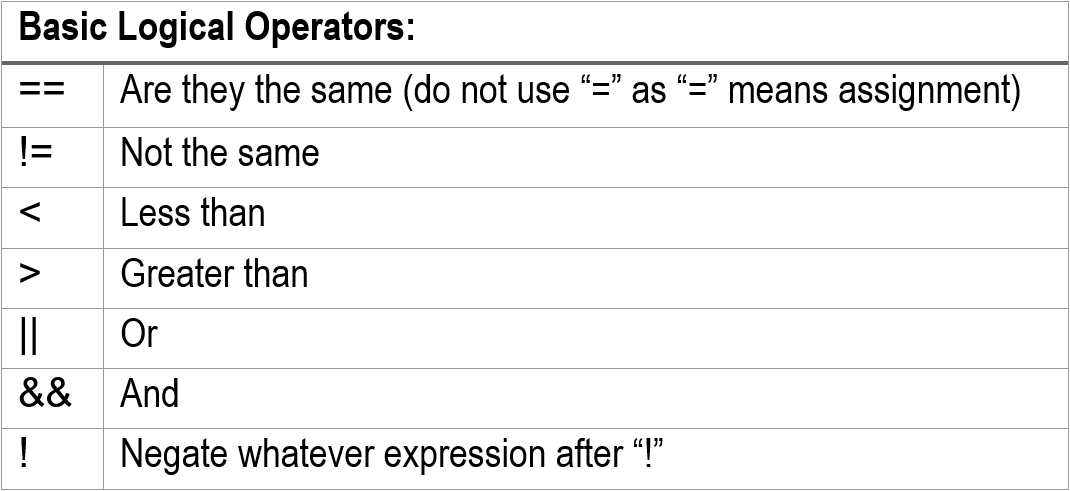# 在 VEXcode Pro V5 中使用布尔值

“bool”数据类型生成逻辑真或假。

##### 逻辑/布尔运算符##### 逻辑/布尔表达式
```if(<boolean expressions>)
{
.....<block>
}
```

`<boolean expressions>` 将包含要计算的单个或复杂表达式. `<block>` 表示只会执行的代码块如果 `<boolean expressions>` 被评估为真。

##### 更多布尔表达式：
 布尔表达式 这是什么意思 如果 (x == 10) 如果 x 等于 10 如果 (x <= 10) 如果 x 小于 且 等于 10 if (x > 10 || y > 20) 如果 x 大于 10 或 y 大于 20 if (x <= 10 && y <= 20) 如果 x<=10 和 y<=20 如果 !(x > 10 || y > 20) 如果 x<=10 和y<=20 if ( !( x <=10 || x >=20) ) 如果 x>10 和x<20

## 使用布尔表达式时要特别小心！

• `if (...)` 将由编译器计算；它产生真或假的含义。
• 真相是：当 ( ... ) 产生 0 以外的任何值（即零）时， `if ( .... )` 表示正确。
• 所以：以下表达式总是正确的：
• 如果 (1)
• 如果 ( 10 )
• 如果（任何结果非零）

```int X = 10, Y=20;
if  (X = Y)
brain.Screen.print("X and Y are the same.”);
else
brain.Screen.print("X and Y are different.”);
```

`如果 (X = Y)`真的意味着：

• 将 Y 分配给 X，因此 X 的值为 20
• 编译器将其解释为 if (20) 其中 (20) 为真，因为它不是 (0)。

```int X = 0, Y=0;
if  (X = Y)
brain.Screen.print("X and Y are the same.”);
else
brain.Screen.print("X and Y are different.”);
```

`如果 (X = Y)` 真的意味着：

• 将 Y 分配给 X，因此 X 的值为 0（零）。
• 编译器将其解释为：(0) 为假。

For more information, help, and tips, check out the many resources at VEX Professional Development Plus